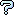All about flooble | fun stuff | Get a free chatterbox | Free JavaScript | Avatarsperplexus dot infoFive Circle Division (Posted on 2006-08-26)Five circles are arranged in the following fashion ( Two rows of 3 circles in square arrangement with one end circle removed from the top row ). Circles are of same diameter and touching adjoining circles as per the diagram. Draw a line passing through A ( Centre of the first circle on the bottom row ) in such a way that it divides the five circles into two equal areas.

```
*                 *
*         *       *         *
*             *   *             *
*               * *               *
*                 *                 *
*               * *               *
*             *   *             *
*         *       *         *
*                 *                 *
*         *       *         *       *         *
*             *   *             *   *             *
*               * *               * *               *
*        A        *                 *                 *
*               * *               * *               *
*             *   *             *   *             *
*         *       *         *       *         *
*                 *                 *

```

 No Solution Yet Submitted by Salil No RatingComments: ( Back to comment list | You must be logged in to post comments.)re(2): Equation spoiler| Comment 8 of 15 |(In reply to re: Equation spoiler by Richard)

With point A at the origin, Larry's equation for the line is correct.

Point B, as it is not in the figure and could be defined as any point of the line, could be at (3,1).  Yet, if point B were to be defined to be a point on the line other than point A, it would be at (3n,1n) where n is a real number other than 0.

A question could be asked, if point A were at origin (0,0) of circle of radius = 1, what are the two points, B and C, on the circles where the line segment BC separates the five circles into areas of equal halves?

 Posted by Dej Mar on 2006-08-26 16:02:15Please log in:

 Search: Search body:
Forums (0)